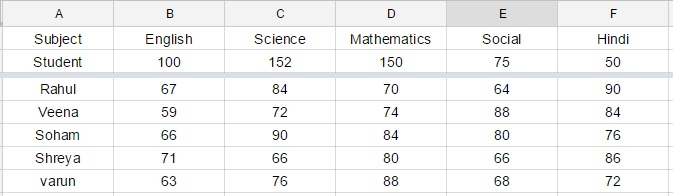IBPS Clerk Data Analysis Test 2

Instructions

Study the given table carefully to answer the question that follow:
percentage of marks obtained by five students in five different subjects in a schoolQ 1

What is the average marks obtained by all the students together in English ?

Q 2

What is varun’s overall percentage in the examination ?

Q 3

What is the ratio of the total marks obtained by Veena and Shreya together in Mathematics to the marks obtained by Rahul in the same subject ?

Q 4

If in order to pass the exam a minimum of 95 marks is needed in Science how many students pass the exam ?

Q 5

What is the total marks obtained by Soham in all the subjects together ?